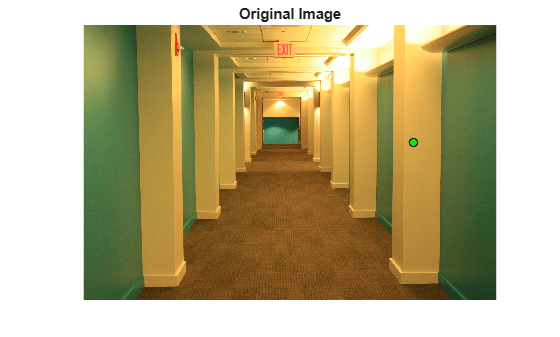## Syntax

``B = chromadapt(A,illuminant)``
``B = chromadapt(A,illuminant,Name,Value)``

## Description

example

````B = chromadapt(A,illuminant)` adjusts the color balance of sRGB image `A` according to the scene illuminant. The illuminant must be in the same color space as the input image.```

example

````B = chromadapt(A,illuminant,Name,Value)` adjusts the color balance of `A` using name-value pairs to control additional options.```

## Examples

collapse all

Read an image with a strong yellow color cast. Display the image, specifying an optional magnification to shrink the size of the displayed image.

```A = imread('hallway.jpg'); figure imshow(A,'InitialMagnification',25) title('Original Image')```Pick a pixel in the image that should look white or gray, such as a point on a pillar. Do not pick a saturated pixel, such as a point on the ceiling light.

```x = 2800; y = 1000; gray_val = [A(y,x,1) A(y,x,2) A(y,x,3)];```

Use the selected color as reference for the scene illumination, and correct the white balance of the image.

`B = chromadapt(A,gray_val);`

Display the corrected image, setting an optional initial magnification.

```figure imshow(B,'InitialMagnification',25) title('White-Balanced Image')```The pillars are now white as expected, and the rest of the image has no yellow tint.

Open an image file containing minimally processed linear RGB intensities.

`A = imread('foosballraw.tiff');`

The image data is the raw sensor data after correcting the black level and scaling to 16 bits per pixel. Interpolate the intensities to reconstruct color. The color filter array pattern is RGGB.

`A = demosaic(A,'rggb');`

Display the image. Because the image is in linear RGB color space, apply gamma correction so the image appears correctly on the screen.

```A_sRGB = lin2rgb(A); imshow(A_sRGB) title('Original Image')```The image has an X-Rite® ColorChecker® chart in the scene. To get the color of the ambient light, display the RGB values of a pixel in one of the neutral patches of the chart. The intensity of the red channel is lower than the intensity of the other two channels, which indicates that the light is bluish green.

```x = 1510; y = 1250; light_color = [A(y,x,1) A(y,x,2) A(y,x,3)]```
```light_color = 1x3 uint16 row vector 7361 14968 10258 ```

Balance the color channels of the image. Use the `'ColorSpace'` option to specify that the image and the illuminant are expressed in linear RGB.

`B = chromadapt(A,light_color,'ColorSpace','linear-rgb');`

Display the color-balanced image with gamma correction.

```B_sRGB = lin2rgb(B); imshow(B_sRGB) title('Color-Balanced Image')```Confirm that the gray patch has been color balanced. The three color channels in the color-balanced gray patch have similar intensities, as expected.

`patch_color = [B(y,x,1) B(y,x,2) B(y,x,3)]`
```patch_color = 1x3 uint16 row vector 13010 13010 13010 ```

## Input Arguments

collapse all

RGB image, specified as an m-by-n-by-3 numeric array.

Data Types: `single` | `double` | `uint8` | `uint16`

Scene illuminant, specified as a 3-element numeric vector. The illuminant must be in the same color space as the input image, `A`.

Data Types: `single` | `double` | `uint8` | `uint16`

### Name-Value Pair Arguments

Specify optional comma-separated pairs of `Name,Value` arguments. `Name` is the argument name and `Value` is the corresponding value. `Name` must appear inside quotes. You can specify several name and value pair arguments in any order as `Name1,Value1,...,NameN,ValueN`.

Example: ```I2 = chromadapt(I,uint8([22 97 118]),'ColorSpace','linear-rgb')``` adjusts the color balance of an image, `I`, in linear RGB color space.

Color space of the input image and illuminant, specified as the comma-separated pair consisting of `'ColorSpace'` and `'srgb'`, `'adobe-rgb-1998'`, or `'linear-rgb'`. Use the `'linear-rgb'` option to adjust the color balance of an RGB image whose intensities are linear.

Data Types: `char` | `string`

Chromatic adaptation method used to scale the RGB values in `A`, specified as the comma-separated pair consisting of `'Method'` and one of:

• `'bradford'`—Scale using the Bradford cone response model

• `'vonkries'`—Scale using the von Kries cone response model

• `'simple'`—Scale using the illuminant

Data Types: `char` | `string`

## Output Arguments

collapse all

Color-balanced RGB image, returned as an m-by-n-by-3 numeric array of the same data type as `A`.